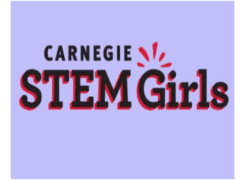### Submit a Resource

Carnegie STEM Girls: MathematicianMathematicians are number crunchers.

Basically, they research and study numbers. Mathematicians can be divided into two groups: theoretical mathematicians and applied mathematicians. Theoretical mathematicians study and prove new mathematical theories. Applied mathematicians use math theories to solve problems in many different fields including business, government, engineering, and the sciences. A doctorate in mathematics is required for most positions in this field.

Read about women who are mathematicians:
Rear Admiral CJ Jaynes
Emmy Noether

Middle School, High School

## Organization

Carnegie STEM Girls

Article